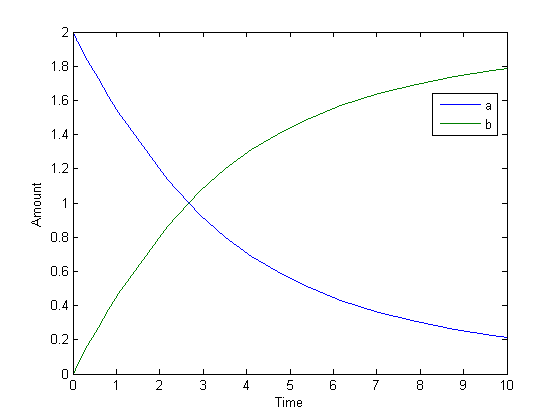Documentation

### This is machine translation

Mouseover text to see original. Click the button below to return to the English version of the page.

# ReactionRate

Reaction rate equation in reaction object

## Description

The `ReactionRate` property defines the reaction rate equation. You can define a `ReactionRate` with or without the `KineticLaw` property. `KineticLaw` defines the type of reaction rate. The `addkineticlaw` function configures the `ReactionRate` based on the `KineticLaw` and the species and parameters specified in the kinetic law object properties `SpeciesVariableNames` and `ParameterVariableNames`.

The reaction takes place in the reverse direction if the `Reversible` property is true. This is reflected in `ReactionRate`. The `ReactionRate` includes the forward and reverse rate if reversible.

You can specify `ReactionRate` without `KineticLaw`. Use the `set` function to specify the reaction rate equation. SimBiology® software adds species variables while creating `reactionObj` using the `addreaction` method. You must add the parameter variables (to the `modelObj` in this case). See the example below.

After you specify the `ReactionRate` without `KineticLaw` and you later configure the `reactionObj` to use `KineticLaw`, the `ReactionRate` is unset until you specify `SpeciesVariableNames` and `ParameterVariableNames`.

For information on dimensional analysis for reaction rates, see How Reaction Rates Are Evaluated .

### Note

If you set the `ReactionRate` property to an expression that is not continuous and differentiable, see Using Events to Address Discontinuities in Rule and Reaction Rate Expressions before simulating your model.

## Characteristics

 Applies to Object: reaction Data type Character vector Data values Character vector defining the reaction rate. Default is `''` (empty character vector). Access Read/write

## Examples

### Add a Reaction Defined by Michaelis-Menten Kinetic Law

Create a model, add a reaction, and assign the expression for the reaction rate equation.

1. Create a model object, and then add a reaction object.

```modelObj = sbiomodel('my_model'); reactionObj = addreaction(modelObj, 'a -> c + d');```
2. Create a kinetic law object for the reaction object of the type `'Henri-Michaelis-Menten'`.

`kineticlawObj = addkineticlaw(reactionObj, 'Henri-Michaelis-Menten');`

`reactionObj` KineticLaw property is configured to `kineticlawObj`.

3. The `'Henri-Michaelis-Menten'` kinetic law has two parameter variables (`Vm` and `Km`) and one species variable (`S`) that you should set. To set these variables, first create the parameter variables as parameter objects (`parameterObj1, parameterObj2`) with names `Vm_d` and `Km_d` and assign them to `kineticlawObj`.

```parameterObj1 = addparameter(kineticlawObj, 'Vm_d'); parameterObj2 = addparameter(kineticlawObj, 'Km_d');```
4. Set the variable names for the kinetic law object.

```set(kineticlawObj,'ParameterVariableNames', {'Vm_d' 'Km_d'}); set(kineticlawObj,'SpeciesVariableNames', {'a'});```
5. Verify that the reaction rate is expressed correctly in the reaction object `ReactionRate` property.

`get (reactionObj, 'ReactionRate')`

MATLAB® returns:

```ans = Vm_d*a/(Km_d + a)```

### Add a Reaction without a Kinetic Law

Create a model, add a reaction, and specify `ReactionRate` without a kinetic law.

1. Create a model object, and then add a reaction object.

```modelObj = sbiomodel('my_model'); reactionObj = addreaction(modelObj, 'a + b -> c + d');```
2. Specify `ReactionRate` and verify the assignment.

```set (reactionObj, 'ReactionRate', 'k*a'); get(reactionObj, 'ReactionRate')```

MATLAB returns:

```ans = k*a```
3. You cannot simulate the model until you add the parameter `k` to the `modelObj`.

`parameterObj = addparameter(modelObj, 'k');`

SimBiology adds the parameter to the `modelObj` with default `Value` = `1.0` for the parameter.

### Define a Custom Hill Kinetic Law that Works with Dimensional Analysis

This example shows how to define a custom reaction rate for the Hill kinetics that is compatible with `DimensionalAnalysis` feature of SimBiology.

This example is useful especially if you are using the built-in Hill kinetic law, but have the kinetic reaction with a non-integer exponent and cannot verify the model because dimensional analysis failed. The built-in Hill kinetic law has the following expression: $\frac{{V}_{m}*{S}^{n}}{{K}_{p}+{S}^{n}}$. Suppose ${K}_{p}={K}_{h}{}^{n}$, then you can rewrite the equation as follows: $\frac{{V}_{m}}{{\left(\frac{{K}_{h}}{S}\right)}^{n}+1}$. The redefined Hill kinetic equation is compatible with Dimensional Analysis and allows you to have a non-integer exponent.

Create a SimBiology model.

`m1 = sbiomodel('m1');`

Add a compartment, two species, and a reaction.

```c1 = addcompartment(m1, 'cell'); s1 = addspecies(m1,'a'); s2 = addspecies(m1,'b'); r1 = addreaction(m1, 'a -> b');```

Add a predefined a Hill kinetic law for the reaction.

`k1 = addkineticlaw(r1, 'Hill-Kinetics');`

Display the rate expression of the built-in kinetic law.

`k1.Expression`
```ans = Vm*S^n/(Kp + S^n)```

Define parameters, values, and units.

```p1 = addparameter(k1, 'Vm', 1.0); p2 = addparameter(k1, 'n', 1.5); p3 = addparameter(k1, 'Kp', 2.828); set(k1, 'ParameterVariableNames', {'Vm','n','Kp'}); set(k1, 'SpeciesVariableNames', {'a'}); set(s1, 'InitialAmount', 2.0); set(s1, 'InitialAmountUnits', 'mole/liter'); set(s2, 'InitialAmountUnits', 'mole/liter'); set(c1, 'CapacityUnits', 'liter'); set(p1, 'ValueUnits', 'mole/liter/second'); set(p2, 'ValueUnits', 'dimensionless'); set(p3, 'ValueUnits', 'mole/liter');```

Verify the model.

`verify(m1)`
```Error using SimBiology.Model/verify --> Error reported from Dimensional Analysis: Dimensional analysis failed for reaction 'a -> b'. When using the power function, both the base and exponent must be dimensionless or the exponent must be an explicit integer constant (for example 2 in 'x^2').```

You are seeing the error message because SimBiology only allows exponentiation of any dimensionless quantity to any dimensionless power.

Redefine the reaction rate so that it is compatible with dimensional analysis and allows a non-integer exponent.

```r1.ReactionRate = 'Vm / ( (Kh/a)^n + 1 )'; k1.KineticLaw = 'Unknown'; ```

Define the value and units for `Kh` parameter.

```p4 = addparameter(k1, 'Kh', 2.0); set(p4, 'ValueUnits', 'mole/liter');```

Verify the model.

`verify(m1)`

You no longer see the error message.

Simulate the model.

`[t,x,names] = sbiosimulate(m1);`

Plot the results.

```plot(t,x); xlabel('Time'); ylabel('Amount'); legend(names);```